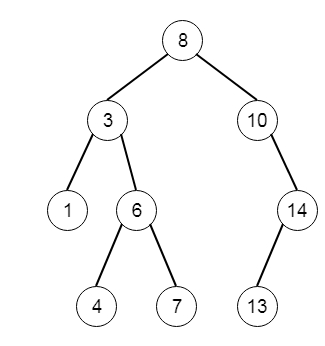# Maximum Difference Between Node and Ancestor in C++

C++Server Side ProgrammingProgramming

Suppose we have the root of a binary tree, we have to find the maximum value V for which there exists different nodes A and B where V = |value of A – value of B| and A is an ancestor of B. So if the tree is like −Then the output will be 7. The ancestor node differences are like [(8 - 3), (7 - 3), (8 - 1), (10-13)], among them the (8 - 1) = 7 is the maximum.

To solve this, we will follow these steps −

• initially define ans 0

• define one method called solve(), this will take the tree node, currMin and currMax. This will act as follows −

• if node is null, then return

• ans := maximum of ans, |value of node - currMin|, |value of node - currMax|

• solve(left of node, minimum of node value and currMin, maximum of node value and currMax)

• solve(right of node, minimum of node value and currMin, maximum of node value and currMax)

• In the main section, call solve(root, value of root, value of root), and return ans

Let us see the following implementation to get better understanding −

## Example

Live Demo

#include <bits/stdc++.h>
using namespace std;
class TreeNode{
public:
int val;
TreeNode *left, *right;
TreeNode(int data){
val = data;
left = NULL;
right = NULL;
}
};
void insert(TreeNode **root, int val){
queue<TreeNode*> q;
q.push(*root);
while(q.size()){
TreeNode *temp = q.front();
q.pop();
if(!temp->left){
if(val != NULL)
temp->left = new TreeNode(val);
else
temp->left = new TreeNode(0);
return;
}else{
q.push(temp->left);
}
if(!temp->right){
if(val != NULL)
temp->right = new TreeNode(val);
else
temp->right = new TreeNode(0);
return;
}else{
q.push(temp->right);
}
}
}
TreeNode *make_tree(vector<int> v){
TreeNode *root = new TreeNode(v);
for(int i = 1; i<v.size(); i++){
insert(&root, v[i]);
}
return root;
}
class Solution {
public:
int ans;
void solve(TreeNode* node, int currMin, int currMax){
if (!node || node->val == 0) return;
ans = max({ans, abs(node->val - currMin), abs(node->val -
currMax)});
solve(node->left, min(node->val, currMin), max(node->val,
currMax));
solve(node->right, min(node->val, currMin), max(node->val,
currMax));
}
int maxAncestorDiff(TreeNode* root) {
ans = 0;
solve(root, root->val, root->val);
return ans;
}
};
main(){
vector<int> v = {8,3,10,1,6,NULL,14,NULL,NULL,4,7,13};
TreeNode *root = make_tree(v);
Solution ob;
cout << (ob.maxAncestorDiff(root));
}

## Input

[8,3,10,1,6,null,14,null,null,4,7,13]

## Output

7